#Function Repository Resource:

# Totatives

Get the integers from 1 to n that have no common proper factor with n

Contributed by: George Beck
 ResourceFunction["Totatives"][n] gives the numbers in the range 1 to n that are relatively prime to n.

## Details and Options

The number of totatives of n is the totient function of n, given by EulerPhi[n].

## Examples

### Basic Examples

The GCDs of these numbers with 10 is 1:

 In:=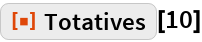Out=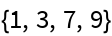In:=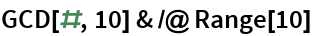Out=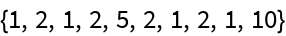In:=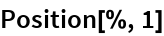Out=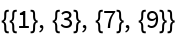In:=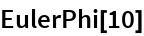Out=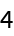This plots the totative of n above (n,0), for n = 1,2,,40:

 In:=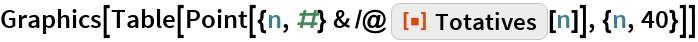Out=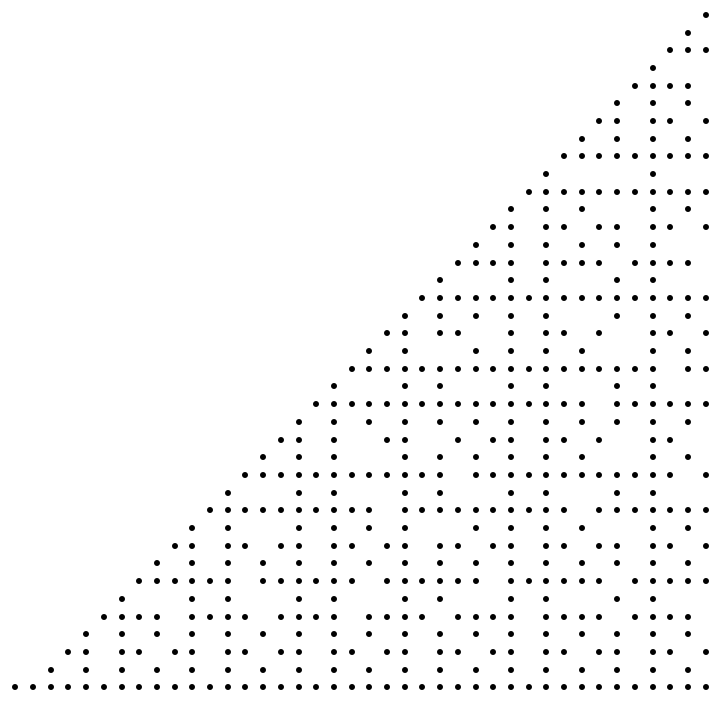### Applications

The totatives of n form a multiplicative group modulo n:

 In:=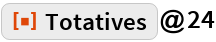Out=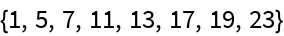Here is its multiplication table:

 In:=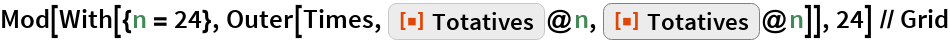Out=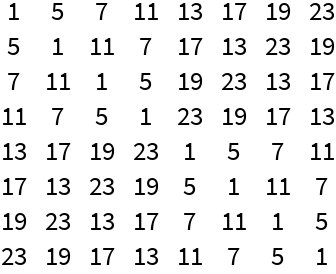## Requirements

Wolfram Language 11.3 (March 2018) or above